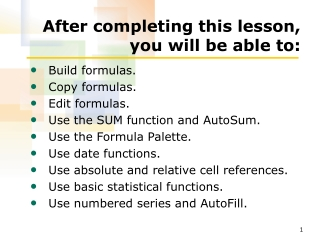DownloadDownload PresentationAfter completing this lesson, you will be able to:

# After completing this lesson, you will be able to:

Télécharger la présentation## After completing this lesson, you will be able to:

- - - - - - - - - - - - - - - - - - - - - - - - - - - E N D - - - - - - - - - - - - - - - - - - - - - - - - - - -
##### Presentation Transcript

1. After completing this lesson, you will be able to: • Build formulas. • Copy formulas. • Edit formulas. • Use the SUM function and AutoSum. • Use the Formula Palette. • Use date functions. • Use absolute and relative cell references. • Use basic statistical functions. • Use numbered series and AutoFill.

2. Building Formulas To enter a formula into a cell • Click the cell and type =. • Type the formula, including cell references, constant values, mathematical operators, functions, and parentheses. • Click the Enterbutton on the Formula bar or press the Enter key.

3. Copying Formulas To copy a formula using the Fill handle • Select the cell that contains the formula that you want to copy. • Drag the Fill handle to the last cell in the desired range.

4. Editing Formulas To revise a formula • Click the cell that contains the formula. • Click the in the Formula bar. • Edit the necessary formula, functions, or arguments and press the Enter key.

5. Deleting Formulas To delete a formula • Click the cell that contains the formula. • Press the Delete key.

6. Using the SUM Function and AutoSum To automatically total a row or column of cells • Select the cell that will contain the total. • On the Standard toolbar, click the AutoSum button. • Modify the arguments, if desired, and press Enter.

7. Inserting Functions To enter a function using the Function Arguments dialog box • Click the cell that will contain the function. • Click the Insert Formula button on the Formula Bar. • Select the function and enter arguments into the Function Arguments dialog box. • Click OK.

8. Using Date Functions To display the serial number that corresponds to a date • Click the cell containing the date. • On the Format menu, click Cells. • In the Format Cells dialog box, change the formatting to the Number category and display the number with zero decimal places.

9. Using the NOW or TODAY Function To use the NOW or TODAY function • Click the cell in which you want the date or date and time to appear. • Type =NOW() or =TODAY() in the cell and press the Enter key.

10. Using Absolute and Relative Cell References To create an absolute reference • Type adollar sign (\$) before the column reference to make the column reference absolute. • Type a dollar sign (\$) before the row reference to make the row reference absolute. • Type a dollar sign (\$) before both the column and row references for a cell to make the entire cell reference absolute.

11. Using Basic Statistical Functions To use basic statistical functions • Check the cell in which you want the function to appear and click the Insert Function button. • Click the Select a function down arrow and click the function that you want to use. • In the Function Arguments dialog box, enter the cells that you want to use in the function and click OK.

12. Working with Numbered Series and AutoFill To use AutoFill • Type the first one or two items of the data pattern into adjacent cells. • Select the range of cells to be filled, including those in the data pattern. • On the Edit menu, point to Fill and click Series. • Click the AutoFill option and click OK.